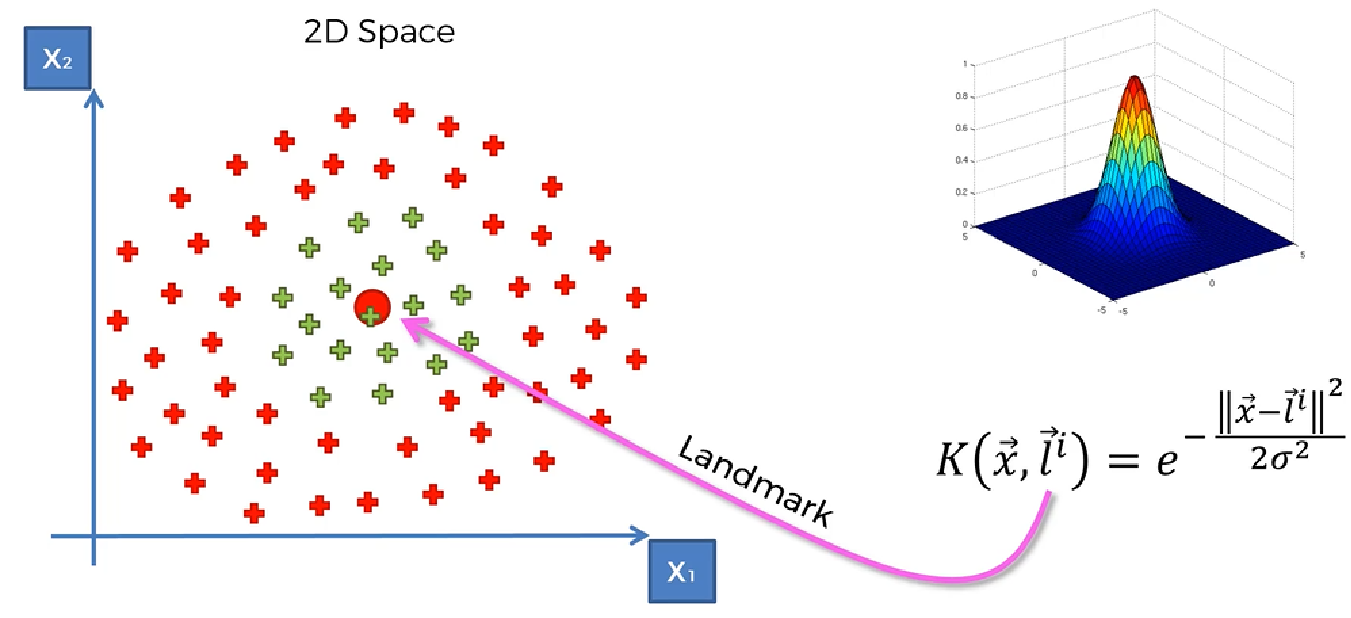# What is Kernel-Support Vector Machine(SVM)?

Original article was published by Manik Soni on Artificial Intelligence on Medium# What is Kernel-Support Vector Machine(SVM)?

## How Kernel-Support Vector Machine(SVM) works? , Why is kernelized SVM much slower than linear SVM?, RBF-kernel SVM from Python’s sklearn library?

Before dive deep into Kernel SVM, we first understand what is Support Vector Machine(SVM)?

So kernel basically in SVM help to create a hyperplane for doing the separation so that we can easily do the categorization, whether it is linearly separable or non-linearly separable.

In linearly separable data, kernel function(linear kernel function) helps to make a hyperplane that can do the separation easily.

But in the case of the dataset where we cannot separate the dataset on 2D plain.

We cannot separate this data linearly

So what strategy we use to separate the dataset

We need to do some analysis on this part. So for that, we need to understand Mapping to a higher dimension. According to this topic when it is difficult to separate the data linearly we need to map it to a higher dimension in order to do the separation.

We try to understand this problem with an example. So let’s take an example

Now we need to separate green and red points as we cannot create a line or linear hyperplane to separate it.

Step 1. Let’s displace points to backward that is 5 points back. So for that, we need to subtract 5 from x variable that is x-5(f=x-5).

Step 2. We know that making the function square of its value creates a U-shape function now the same thing we apply on this function that is (x-5)²

Step 3. On projecting the value on the U-shape function.

Step 4. Now if we draw a linear line it helps to separate the dataset.

This concept is known as Mapping to higher dimensions wherein the above example we map 1D to 2D.

Now, our question is how to separate this dataset?

So, similarly, we have to do the mapping to a higher dimension.

For this, we have used the Gaussian RBF Kernel function.

In the above equation:

‘K’ stands for Kernel Function

‘x’ stands for data points

‘l’ stands for landmark

‘σ’ stands for the circumference of separation.

The above picture gives you a description of how the Kernel looks like for the separation?

So, now let’s see where is a landmark?

This landmark is put in the middle of a separation.

Now if a point is far away from this landmark, it is located on the below plain, and the value of kernel function is zero(0) because you can see the power of ‘e’(exponent), is bigger if the difference from the landmark is bigger and the more negative the power of ‘e’(exponent) which means it values is near to zero.

If a point is near to the landmark then the value of the power of ‘e’(exponent) is near to zero then the value of kernel function value is one(1).

Now, with this concept, we can solve the problem of 2D dataset.

Now, put the landmark and do hyperplane separation.